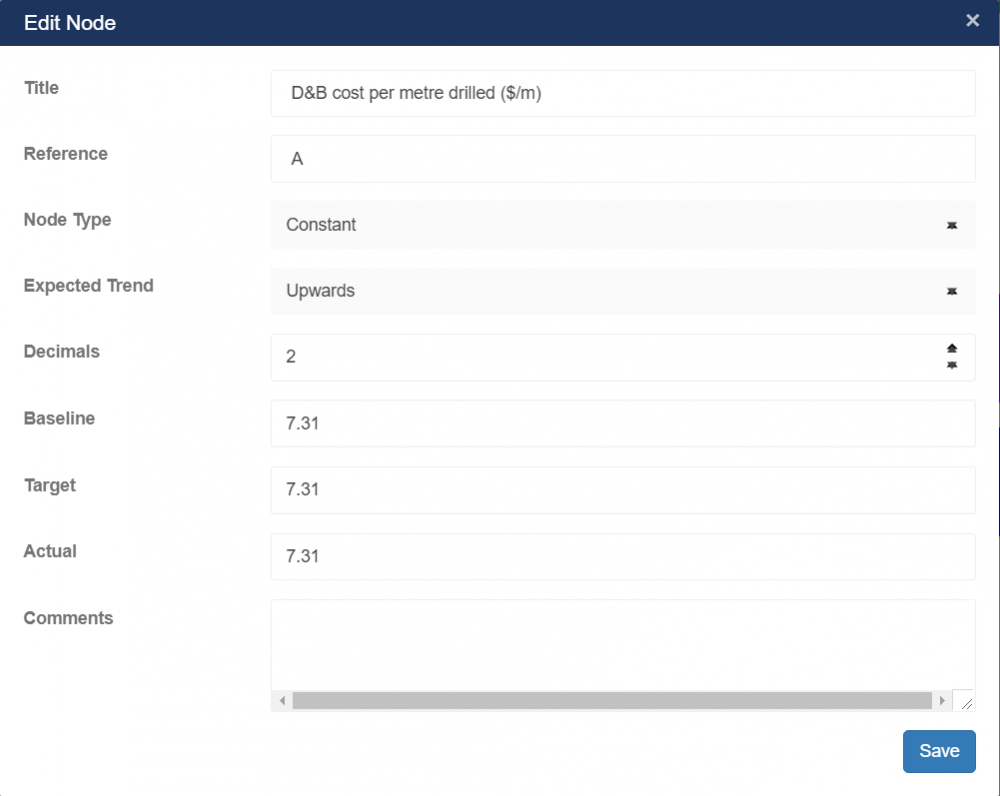Node Overview

Each node in the VDT contains information, as well as controls to modify the node or the VDT. The colour of the node is determined by the type of the node (Parent, KPI or Constant), with the colours configured on the Site Settings Page.1. The name of the Node, as set up in the Node Edit Dialog
2. Collapses/expands all the children of this node
3. Contains the list of fields, as set up in the VDT Edit Page, as well as their values.
• These values are either calculated, read from the input captured in the Node Edit Dialog or retrieved from the KPI that the node is associated with
4. Indicates that this node’s values are calculated and shows the formula used in the calculation.
5. Provides node edit functionalities detailed as follows:
• First button, Opens the Create Node Dialog to allow for the creation of child nodes
• Second button, Opens the Edit Node Dialog
• Third button, Removes the current node from the VDT

Edit Node• Title
• This value will be displayed at the top of the node and is used to identify/describe the node
• Reference
• A unique identifier for this node
• To be used in the expression of the parent node
• Must start with an alphabetic character and no spaces are allowed
• Automatically allocated a value from a – z, based on the node’s position, but can be changed to any other valid identifier for ease of use
• Node Type
• Parent Node
• Indicates that this node will have further child nodes
• Allows for input of an expression to calculate the node’s value based on child node values
• Input KPI
• Indicates that this node’s value is derived from a KPI
• Exact KPI values are determined by the KPI selected for the node, as well as the start/end date range of each field
• Constant
• Indicates that this node’s value is a fixed (constant) value
• Values are entered into the “Input Fields” section below
• Variable Value
• Indicates that this node’s values are fixed values.
• This type of node allows you to specify all the values including the actual value and acts more like a constant KPI node(actual value captured will never change)
• VDT Value
• Indicates that this node’s value is derived from another VDT.
• This type of node allows you extract the field values from another VDT.
• KPI
• The KPI to be used to read this node’s value from
• Expression
• The expression to be used to calculate the field values for this node
• Based on a combination of child node references, expressions and functions
• Decimals
• The number of decimal places to be used when displaying this node’s values
• Input Fields
• The list of baseline and target fields, as set up on the Create/Edit VDT Page
• Can be used for any comments, descriptions or other free-text information relevant to this node

Depending on the type of the node (selected in the Node Type dropdown), different input fields will be displayed within this dialog. The complete list of fields are listed below.

Node Expressions

Expressions are used to specify how a field or child node calculates its value. These can be made up of any combination of the following:

• References to other fields (for calculated fields) or child nodes (for calculated nodes)
• Constant values
• Function calls

For example, the following is a valid expression: pow(a, 2) * avg(a, b) / abs(min(a * 50, b))

In this case:

• a and b are references to other fields or nodes
• 2 and 50 are constant values
• pow, abs and min are function calls

During evaluation of the expression, references are replaced with their appropriate values (from the field/node it referenced) and the expression is then evaluated using the standard mathematical order of operations:

1. brackets
2. function calls
3. multiply/divide operations

### The following functions are currently supported:

FunctionDescriptionExample Usage
absReturns the absolute value of a numberabs(a)
ceilReturns the smallest integral value that is greater than or equal to the specified numberceil(a)
floorReturns the largest integer less than or equal to the specified numberfloor(a)
roundRounds a number to the nearest integral valueround(a)
truncReturns the integral part of a specified numbertrunc(a)
expReturns e raised to the specified powerexp(a)
minReturns the smaller of two numbersmin(a, b)
maxReturns the larger of two numbersmax(a, b)
avgReturns the average of two numbersavg(a, b)
powReturns a specified number raised to the specified powerpow(a, b)
sqrtReturns the square root of a specified numbersqrt(a)
logReturns the natural (base e) logarithm of a specified numberlog(a)
log10Returns the base 10 logarithm of a specified numberlog10(a)
sinReturns the sine of the specified anglesin(a)
cosReturns the cosine of the specified anglecos(a)
tanReturns the tangent of the specified angletan(a)
asinReturns the angle whose sine is the specified numberasin(a)
acosReturns the angle whose cosine is the specified numberacos(a)
atanReturns the angle whose tangent is the specified numberatan(a)
atan2Returns the angle whose tangent is the quotient of two specified numbersatan2(a, b)
sinhReturns the hyperbolic sine of the specified anglesinh(a)
coshReturns the hyperbolic cosine of the specified anglecosh(a)
tanhReturns the hyperbolic tangent of the specified angletanh(a)